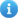COVID-19 | Information and resources to help

# Depreciation

Each Stockitem Stats code is associated with a Group. These groups are used to assign depreciation rates and cost prices to items. The actual prices used are formulated by STATE LIBRARY OF WESTERN AUSTRALIA. By Depreciating by Groups varying percentage depreciation figures can be set, and Local stock can be exempt from depreciation as required. Depreciation can be set via Location or for All Locations using [F8] for specific Location and [F9] for All Locations.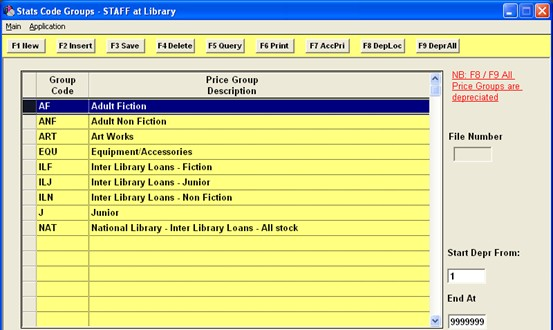To view Price information for any of the Stats groups, highlight the line and select AccPri [F7]. A table will display, which allows the entry of current depreciation rates and minimum prices for each Form.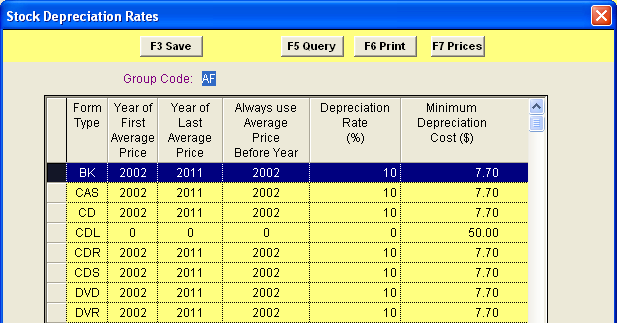COLUMN

EXPLANATION

Form Type

Available Form Types that can be allocated average prices

Year of First Average Price

First average price as defined on the Prices Table for this particular form.

Year of Last Average Price

Last average price as defined on the Prices Table for this particular form.

Always use Average Price Before Year

Before the year entered in this column, give the average price for the particular year rather than calculate according to percentage

Depreciation Rate (%)

The rate at which the prices will be depreciated in the new calculation

Minimum Depreciation Cost (\$)

A minimum amount, so that if the value of the calculation falls below this amount, enter this amount in the cost field rather than the calculated figure

To be eligible for depreciation, depreciation rates must be entered for every type of Form.

In addition, an average price for each year can be entered for Forms of items by selecting Prices [F7].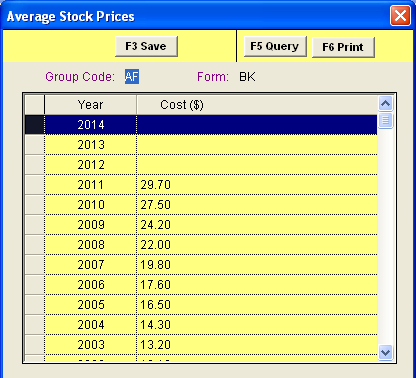## Running Depreciation

Depreciation can be set to be depreciated on a Straight Line basis or on a Reducing Balance basis. The method is selected in Supervisor, Library Menu, Installation, Stockitem – Deprec. Items on

straight line basis. An option as to whether 10% GST is removed prior to depreciation and then reapplied can be selected from Supervisor, Library Menu, Installation, System – If price includes GST this removed before depr and recalculated after. Regardless of the Depreciation method, the same Depreciation Tables are used within Amlib. The essential difference is in the calculation and which cost price is used for the calculation.

The Depreciation can be performed on one item or as a Mass Depreciation from Stockitem, StatsGroups.

The date Depreciation uses is the Accession Date of the Stockitem. If there is no Accession Date, it uses the Received Date of the Stockitem.

As the item is depreciated, the system considers:

Method of Depreciation e.g. Straight Line or Reduced Balance

The Stats Group code which has the Percentage Rate, Minimum price restrictions

Last Depreciation date (items Depreciated in the last 12 months will not be included or new items accessioned in the current year will not be subject to Depreciation)

Rounding formulas as set in Stockitem, Application, StockOrigins for the particular Source

## Straight Line Depreciation

A Straight Line Depreciation uses the Original Cost Price which is stored in Stockitem/Item/History.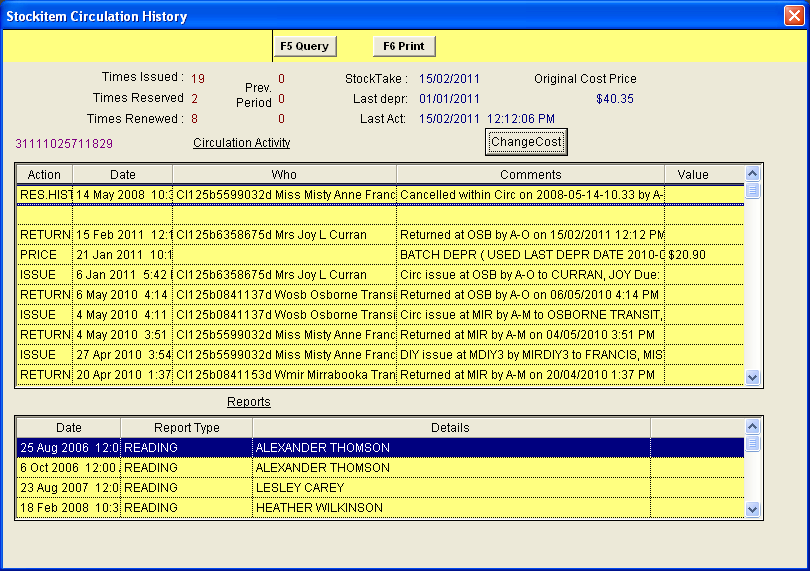Note: The Original Cost field is assigned the same value as the cost field when a new item is manually inserted Example or Straight Line Depreciation

An item worth \$27.00 was purchased in 1995, and therefore 6 years old at 2001. Within the tables, the depreciation rate is set at 10%

It simply calculates 60% (6 years x 10% = 60%) of the Original Cost e.g. of \$27.00 This will be \$16.20

So the Depreciated pricing will be \$27-\$16.20= \$10.80, rounded off will be \$11.00

Note: Rounding parameters are set in Stockitem, Application, StockitemOrigins

## Reducing Balance

A Reducing Balance the current cost (i.e. the value visible on the Stockitem Window) is used.

Example or Reducing Balance Depreciation

An item worth \$27.00 was purchased in 1995, and therefore 6 years old at 2001. Within the tables, the depreciation rate is set at 10%

• 1st year it depreciates to \$24.30
• 2nd year it depreciates to \$21.87 (\$24.30 – 2.43)
• 3rd year it depreciates to \$19.69 (\$21.87 – 2.18)
• 4th year it depreciates to \$17.73 (\$19.69 – 1.96))
• 5th year it depreciates to \$15.96 (\$17.73 - 1.77)
• 6th year it depreciates to \$14.37 (\$15.96 – 1.59) Rounded off that will be \$14.00

## Depreciation Process

The Depreciation process can take a large amount of system resources to implement, and should not be done when the library is busy as all other applications will be slow

Items can be depreciated on a yearly basis. Depreciate all items at the Login Location via the Stats Group Table by selecting DepLoc [F8]. To depreciate all items use DepAll [F9].

## Start Depr From, End At

The value in this field is the unique Stockitem number and depending upon your installation could be in the range 1 to 999999 depending upon how many items are in the database.

A message will display: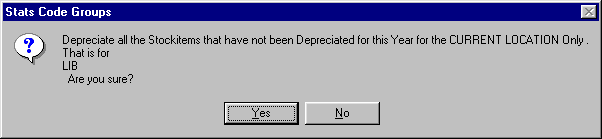For every item on the database at the Current Location this will

Assign an average price if the price is 0.00 and a parameter entry can be found

Depreciate the current price by the respective % if the price has not be depreciated within the last 12 months (a field in the Stockitem History records the last depreciation date).

At the end of the process, a message will display.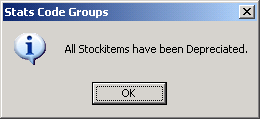The Accession date of an item is used in these calculations.

Note: New items accessioned within the last 12 months will not be depreciated

The Stockitem history will keep a note of the Depreciation change and enters the Last Depreciation Date.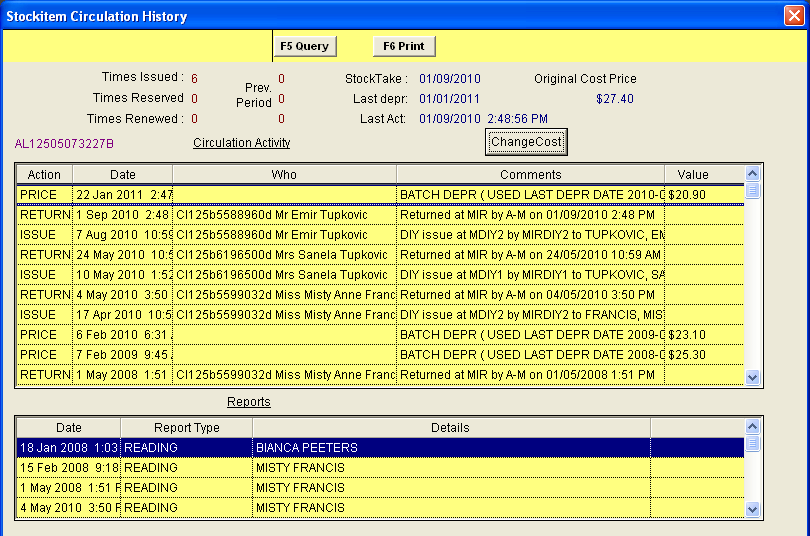Individual items can also be depreciated from Stockitem, Item, Depreciate item.